• 里面有MATLAB基础的案例，非常的全面，适合MATLAB的初学者
• ## matlab例题

千次阅读 2018-04-03 15:52:09
这个题我先用的两个参数画的两个图形，但是我真不会用相同的参数把交线表示出来，问了问老师最后是暴力跑一遍点画的r=linspace(0,1,100); t=0:pi/50:2*pi; [R T]=meshgrid(r,t); x=R.*cos(T); y=R.*sin(T);...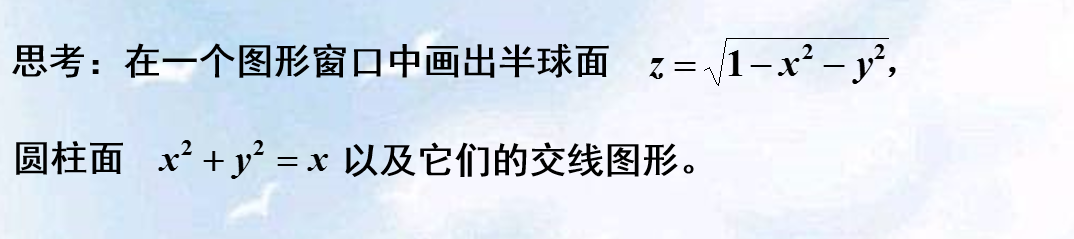这个题我先用的两个参数画的两个图形，但是我真不会用相同的参数把交线表示出来，问了问老师最后是暴力跑一遍点画的r=linspace(0,1,100);
t=0:pi/50:2*pi;
[R T]=meshgrid(r,t);
x=R.*cos(T);
y=R.*sin(T);
z=sqrt((1-x.*x - y.*y)+eps);
mesh(x,y,z);
axis equal
hold on;
z1=R;
x1=cos(T).^2;
y1=sin(T).*cos(T);
mesh(x1,y1,z1);
shading flat
[x y]=meshgrid(-1:.002:1);
z=sqrt((1-x.*x - y.*y)+eps);
for i=1:1:1000
for j=1:1:1000
if((1-x(i,j).*x(i,j) - y(i,j).*y(i,j))>=0)
if(abs(x(i,j)*x(i,j)+y(i,j)*y(i,j)-x(i,j))<=1e-3)
plot3(x(i,j),y(i,j),sqrt((1-x(i,j).*x(i,j) - y(i,j).*y(i,j))),'b*');
end
end
end
end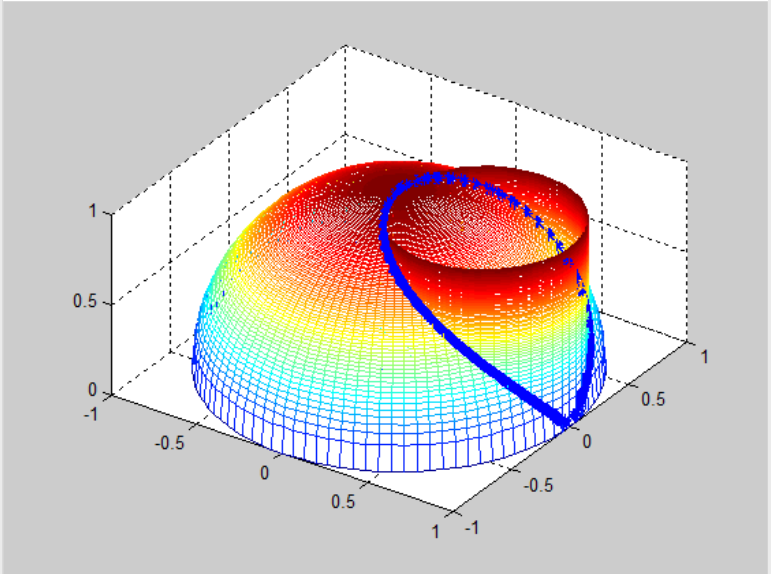修改一下以上做法，实在是有点傻，把T带进去表示出来就完了，我怎么就想不起来呢，一直纠结那个R了r=linspace(0,1,100);
t=0:pi/50:2*pi;
[R T]=meshgrid(r,t);
x=R.*cos(T);
y=R.*sin(T);
z=sqrt((1-x.*x - y.*y)+eps);
mesh(x,y,z);
axis equal
hold on;
z1=R;
x1=cos(T).^2;
y1=sin(T).*cos(T);
mesh(x1,y1,z1);
shading flat
x=cos(T).*cos(T);
y=cos(T).*sin(T);
z=(1-x).^0.5;
plot3(x,y,z,'bh','linewidth',5);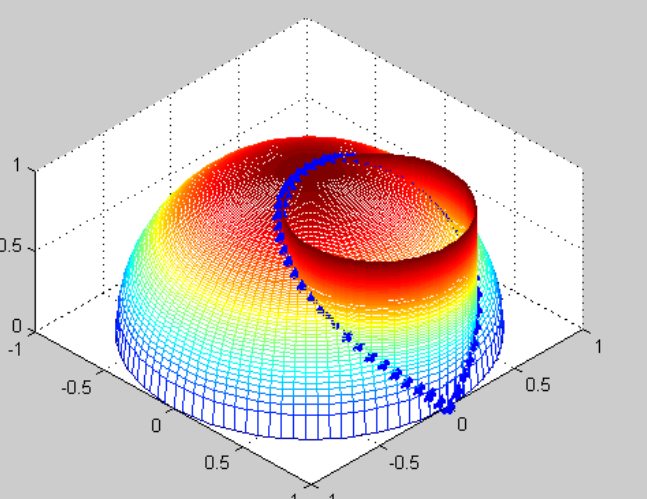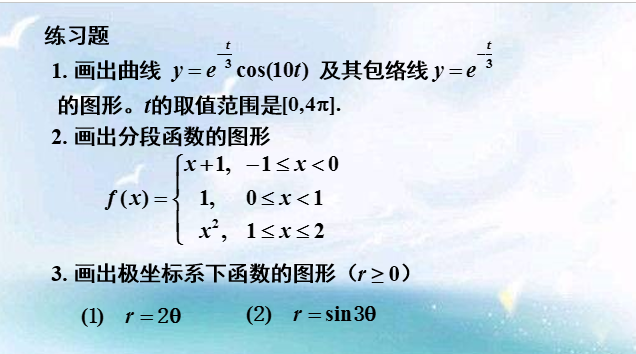1.t=0:pi/50:4*pi;
x=exp(t./3).*cos(10.*t);
y=exp(t./3);
plot(t,x,'g',t,y,'r');2.x=-1:.001:2;
y=(x+1).*(x>=-1 & x<0)+(x>=0 & x<1) +x.^2.*(x>=1 & x<2);
plot(x,y)3.极坐标下画图我有点疑问，在为西塔的奇数倍时，函数都能画出来，偶数倍的就要先算下角度大小使R为正t=[-4*pi/3:pi/90:-pi,-2*pi/3:pi/90:-pi/3,0:pi/90:pi/3, pi*2/3:pi/90:pi];
% t=0:pi/100:10*pi;
r=sin(3*t);
polar(t,r,'g')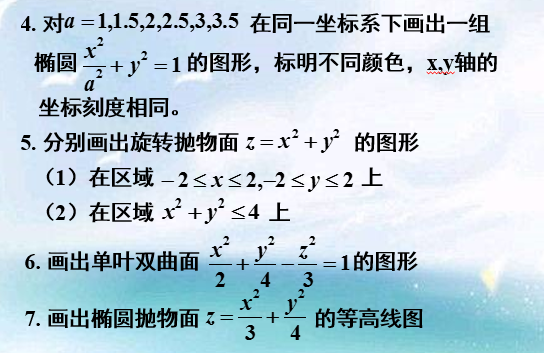4.a=[1,1.5,2,2.5,3,3.5];
l=length(a);
color1=['r','g','b','y','m','c'];
t=-2*pi:pi/50:2*pi;
hold on;
for i=1:1:l;
plot(a(i).*cos(t),sin(t),color1(i));
end5.[x,y]=meshgrid(-2:.01:2);
z=x.^2+y.^2;
surf(x,y,z);
shading flat在范围内t=0:pi/40:pi*2;
r=0:0.01:2;
[R,T]=meshgrid(r,t);
x=R.*cos(T);
y=R.*sin(T);
z=x.^2+y.^2;
surf(x,y,z)
shading flat6.clc
clear all
[x,y]=meshgrid(-3:.01:3);
z1=sqrt((3/2)*x.*x +3*y.*y-3);
z2=-sqrt((3/2)*x.*x +3*y.*y-3);
surf(x,y,real(z1));
hold on
surf(x,y,real(z2));
shading flat上面的求法是错误的，因为取x y的范围不对，但是为了日后复习知道怎么错的，还是保留一下，出现了复数，其他的方法也没想到，就用参数方程好了a=sqrt(2);
b=sqrt(3);
c=2;
[t f]=meshgrid(-2*pi:pi/100:2*pi);
x=a*sec(t).*cos(f);
y=b*sec(t).*sin(f);
z=c*tan(t);
mesh(x,y,z)
shading flat现在给出另一种画法，将取的参数改变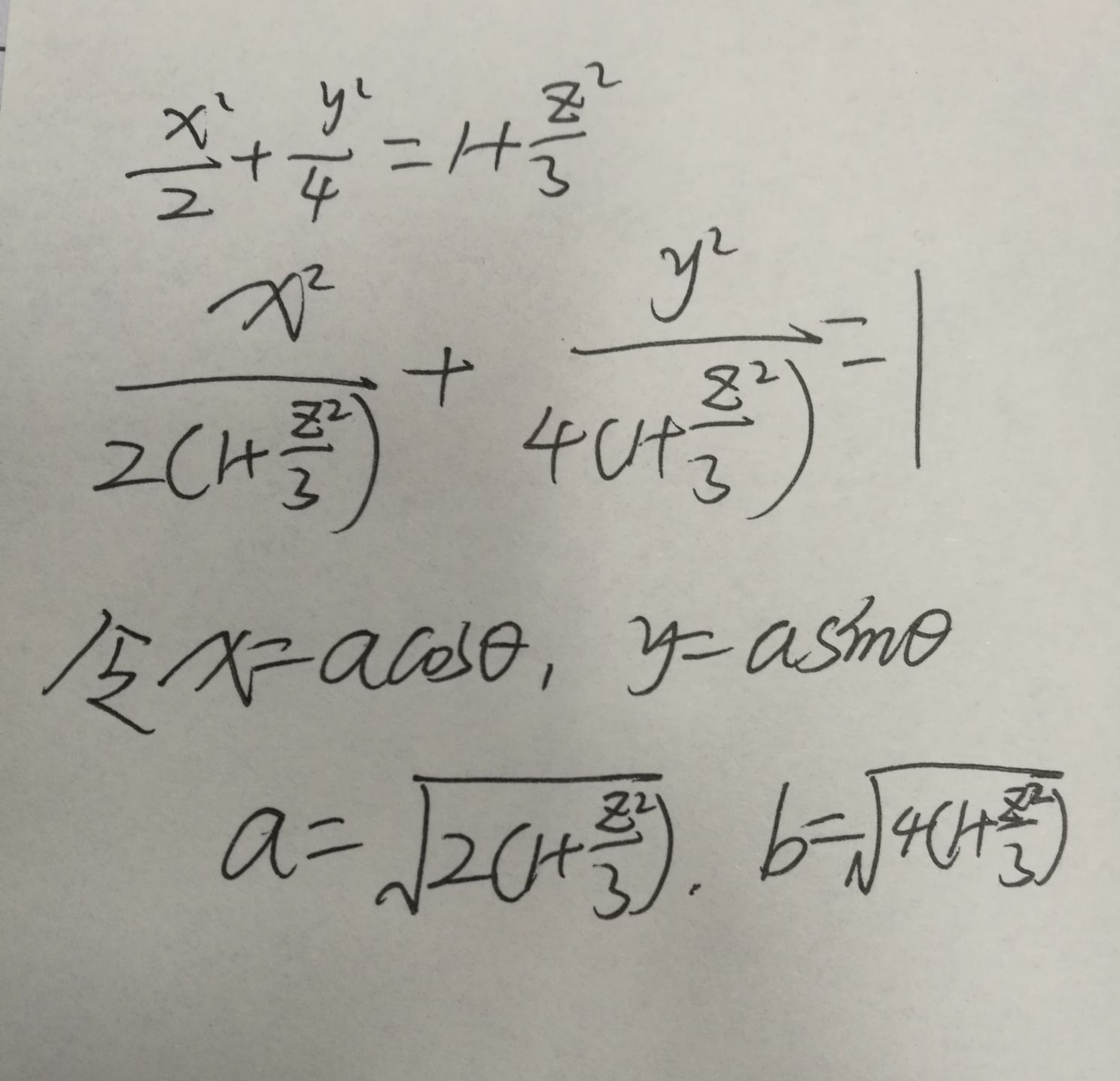z=-5:0.01:5;
t=0:pi/50:2*pi;
[Z,T]=meshgrid(z,t);
x=sqrt(2*(1+Z.*Z/3)).*cos(T);
y=sqrt(4*(1+Z.*Z/3)).*sin(T);
surf(x,y,Z)
shading flat7.[x,y]=meshgrid(-2:.01:2);
z=x.^2/3+y.^2/4;
subplot(2,2,1);
mesh(x,y,z);
subplot(2,2,2);
[C,h]=contour(z,6);
axis equal
clabel(C,h)
subplot(2,2,3);
[C,h]=contourf(z,6)
clabel(C,h);
展开全文• MATLAB例题考试及答案· 里面有经典MATLAB例题考试及答案
• 请将下面给出的矩阵A和B输入到MATLAB环境中，并将它们转换成符号矩阵。若某一矩阵为数值矩阵，另以矩阵为符号矩阵，两矩阵相乘是符号矩阵还是数值矩阵。
• MATLAB程序设计与应用（第二版）刘卫国主编，其中有书中所有例题的源程序
• 牛顿迭代算法 matlab 程序 1 牛顿迭代法描述 牛顿法求实系数高次代数方程 f(x)=a0x^n+a1x(n-1)++an-1x+an= an 1 的在初始值x0 附近的一个根 解非线性议程 f(x)=0 的牛顿法是把非线性方程线性化的一种近似方法 把 f(x...
• z(2)e xyz程序：syms x y z; f=exp(z)-x*y*zzx=-diff(f,x)/diff(f,z) zy=-diff(f,y)/diff(f,z)运行结果：>> lx372 f =exp(z) - x*y*z zx =(y*z)/(exp(z) - x*y) zy =(x*z)/(exp(z) - x*y) 8....
z(2)e xyz程序：syms x y z; f=exp(z)-x*y*zzx=-diff(f,x)/diff(f,z) zy=-diff(f,y)/diff(f,z)运行结果：>> lx372   f =exp(z) - x*y*z     zx =(y*z)/(exp(z) - x*y)     zy =(x*z)/(exp(z) - x*y) 8.证明函数u ln(x a)2 (y b)2(a,b为常数)满足拉普拉斯方程:2u 2u022 x y(提示：对结果用simplify化简)练习4 积分计算x2dx x 1       (2)sin2xdx sin21.计算下列不定积分.     (1)2.计算下列定积分.     (1) 1txexlnxdx(2)3 4xsin2xdx1 lnx 1(xlnx)2dx3.求并用diff对结果求导.4.求摆线x a(t sint),y a(1 cost)的一拱(0 t 2 )与x轴所围成的图形的面积.5.计算二重积分(x(1)x2 y2 16.计算Ly)dxdy(x2(2)x2 y2 x2y2)dxdyx2 y2dsL为圆周x y2 ax(a 0)
展开全文• 1.3-4 MATLAB提示出现错误 1.3-5 用函数 zeros生成全零阵 1.3-6 用函数eye生成全零阵 1.3-7 矩阵的加减运算 1.3-8 两个矩阵的乘法运算 1.3-9 矩阵的数乘运算 1.3-10 向量的点积 1.3-11 向
1.3-2 输入一个行矩阵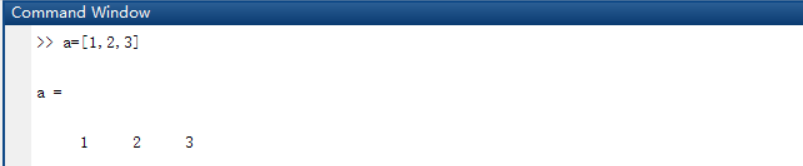1.3-3 可以分行输入一个行矩阵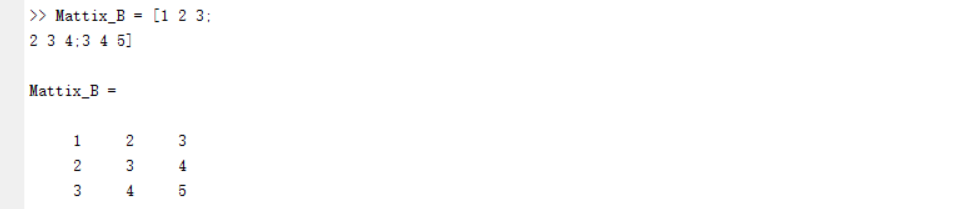1.3-4 MATLAB提示出现错误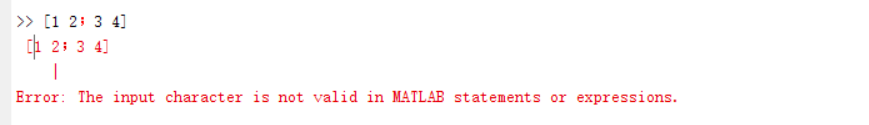1.3-5 用函数 zeros生成全零阵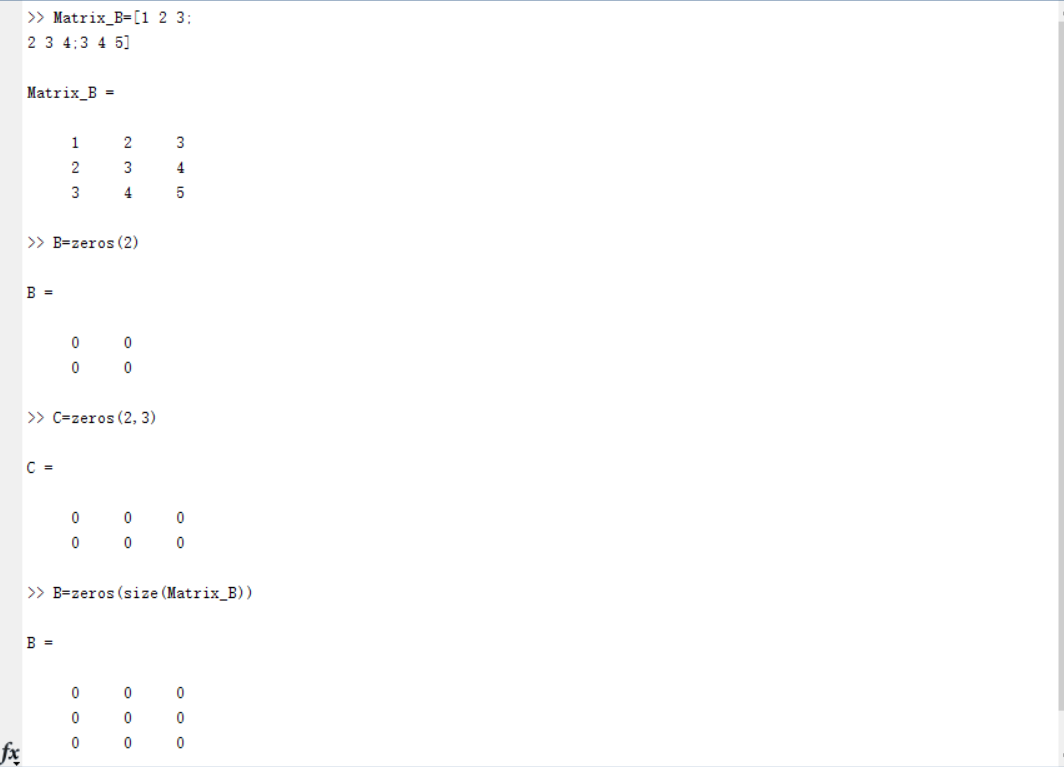1.3-6 用函数eye生成全零阵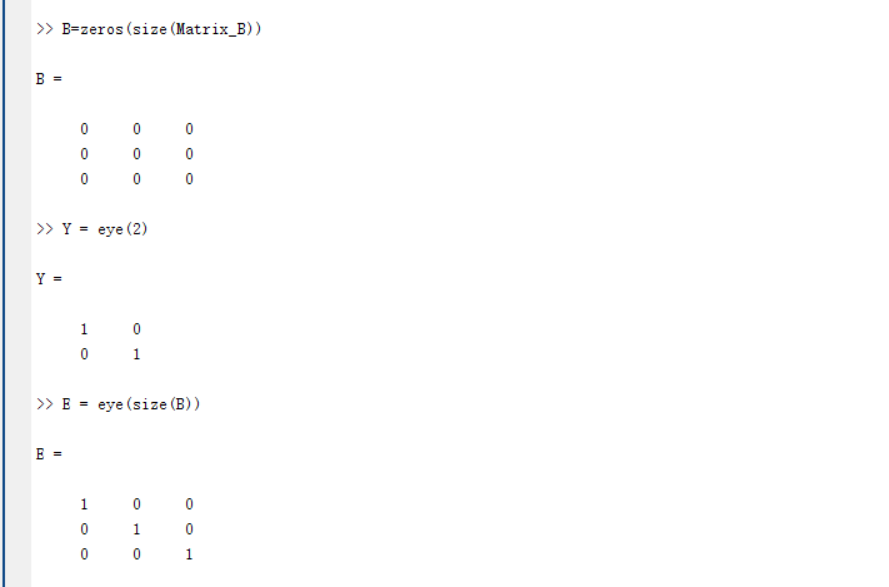1.3-7 矩阵的加减运算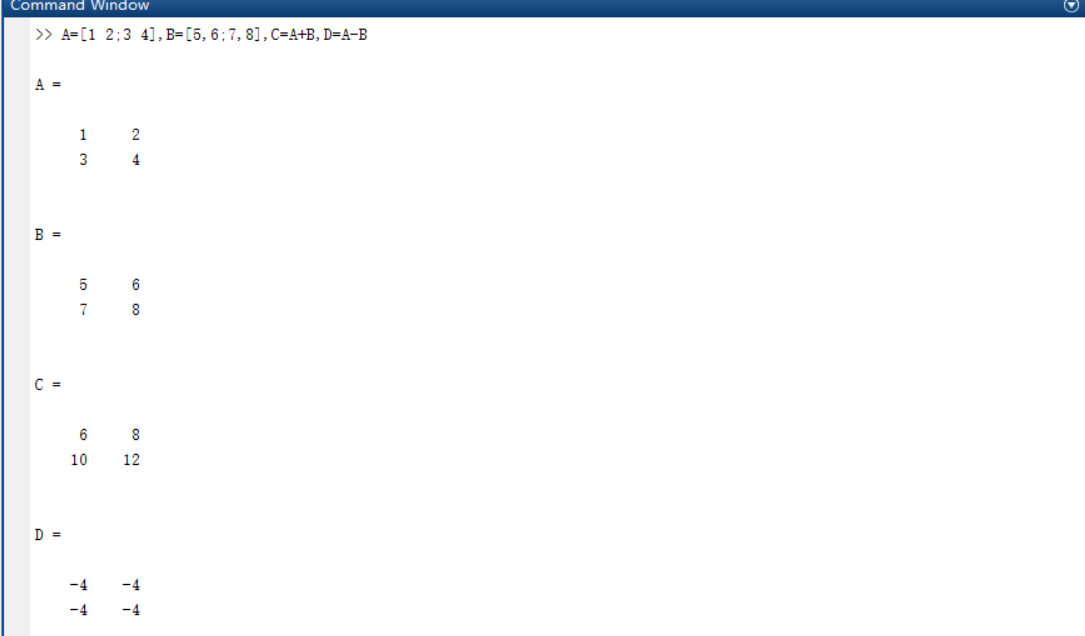1.3-8 两个矩阵的乘法运算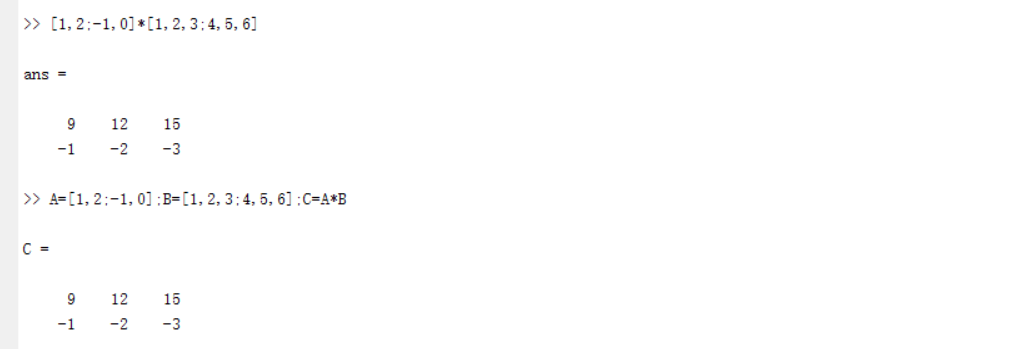1.3-9 矩阵的数乘运算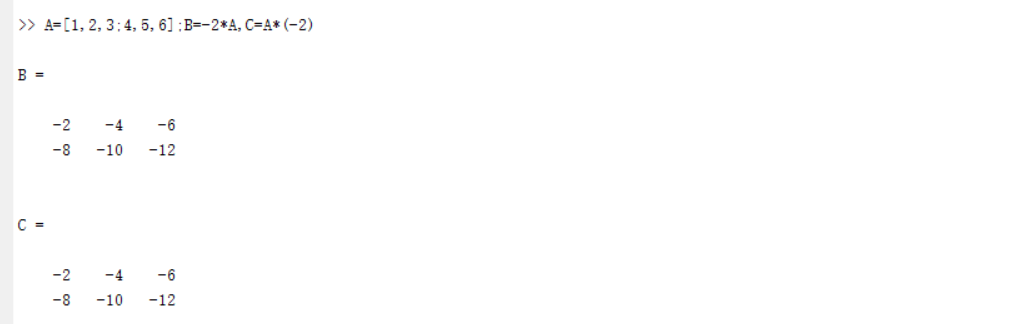1.3-10 向量的点积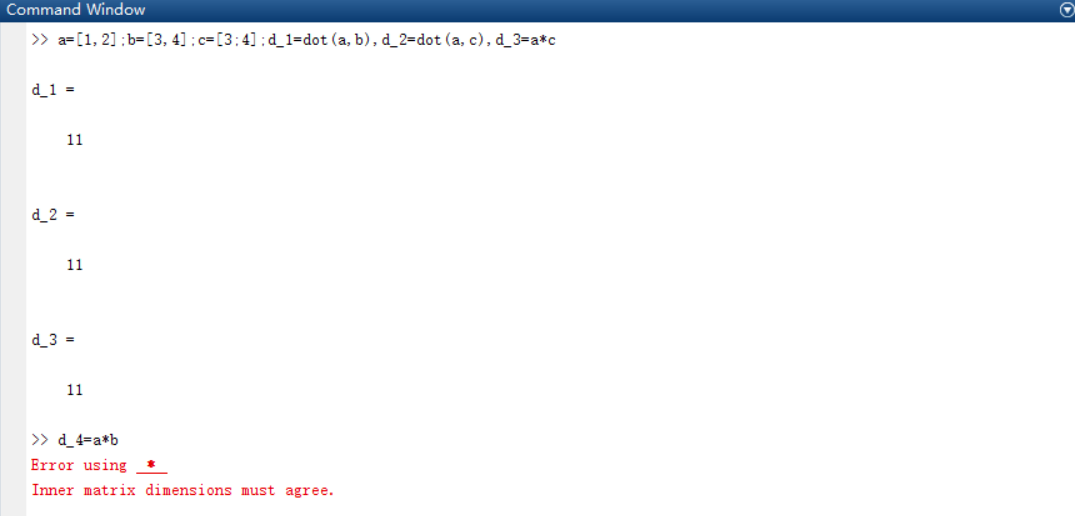1.3-11 向量的叉乘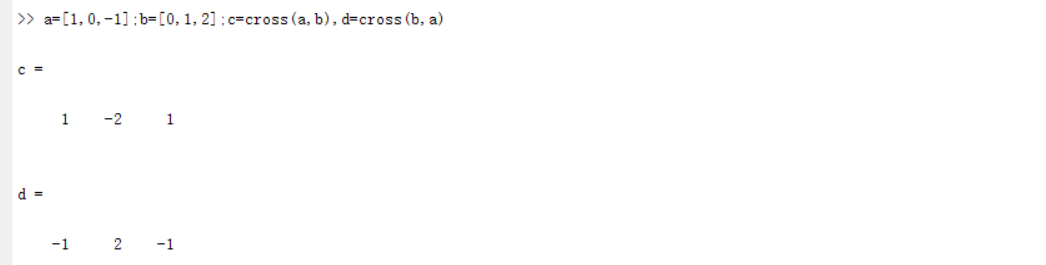1.3-12 向量的混合乘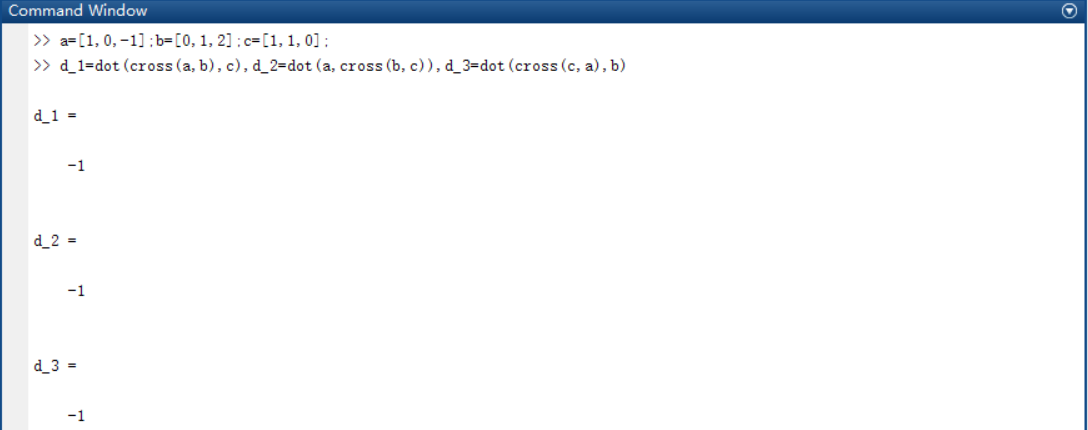1.3-13 左乘和右乘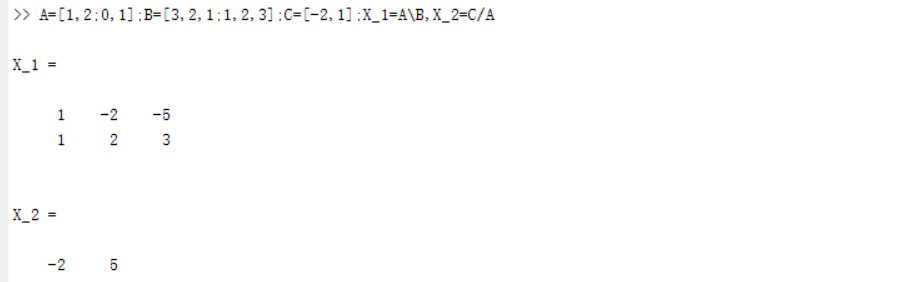1.3-14 矩阵的乘方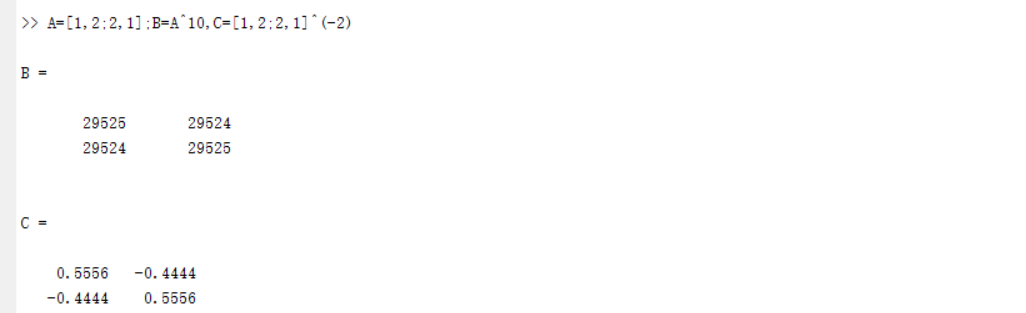1.3-15 矩阵的转置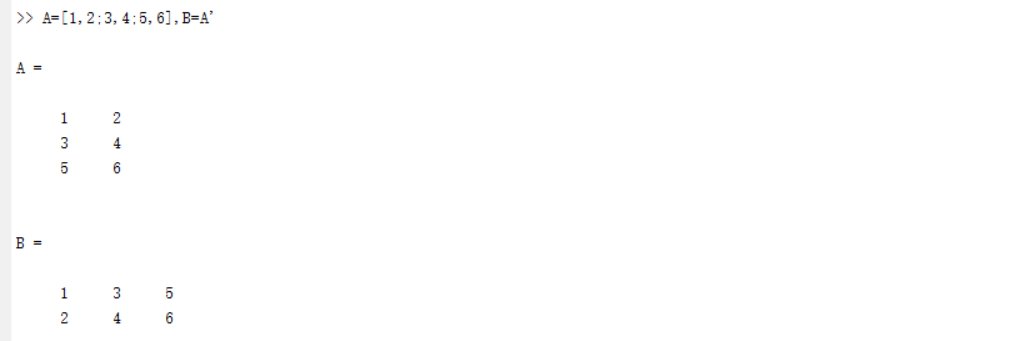1.3-16 对奇异矩阵求逆时MATLAB给出的警告信息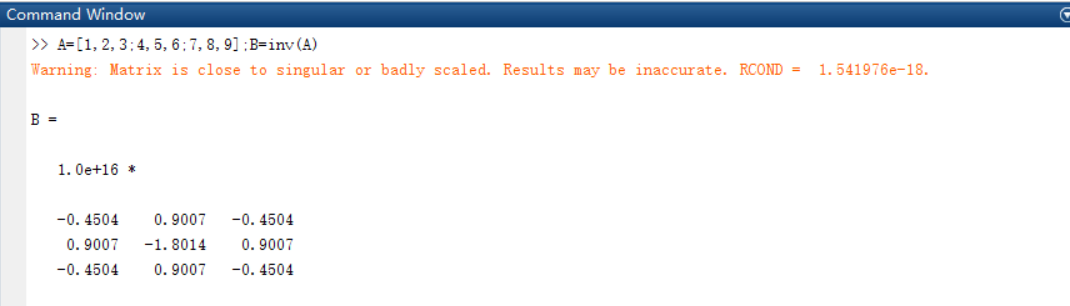1.3-17 用初等变换的方式求逆矩阵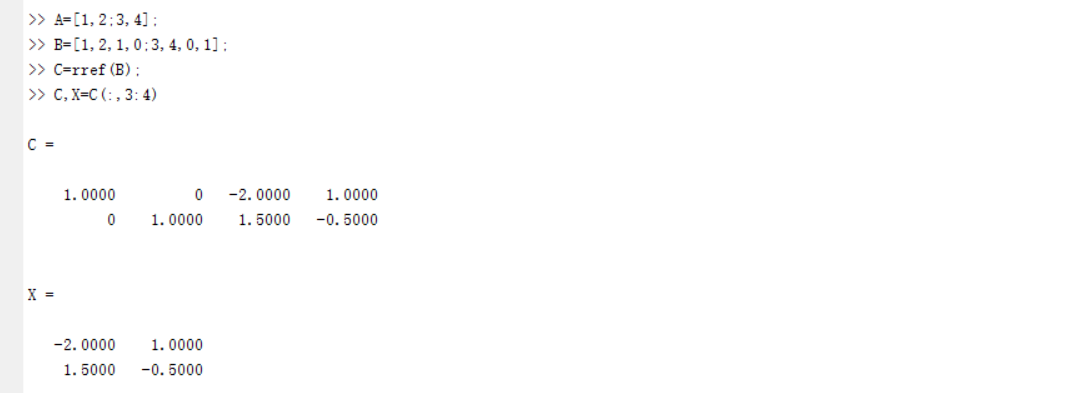1.3-18 以有理格式输出结果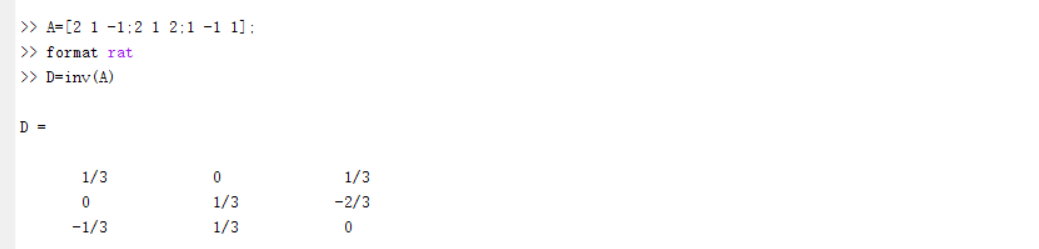demo1：稀疏矩阵的应用以及图形和矩阵之间的关系
图形是一组节点，它们之间具有指定的连接。一个例子是Buckminster Fuller测地圆顶（也是足球或碳-60分子）的连通图。在MATLAB中，测地圆顶图可以用BUCKY函数生成
％定义变量。
[B，V] =巴基;
H =稀疏（60,60）;
k = 31:60;
H（k，k）= B（k，k）;
％可视化变量。
gplot（B-H，V，'b-'）;
等一下
gplot（H，V，'r-'）;
拖延
轴关闭相等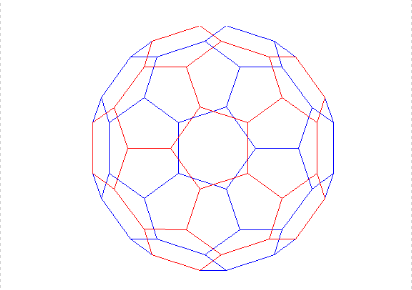可以通过其邻接矩阵来表示图。为了构造邻接矩阵，节点被编号为1至N.然后，如果节点i连接到节点j，则矩阵的元素（i，j）被设置为1，否则为0 。
％定义矩阵A.
A = [0 1 1 0; 1 0 0 1; 1 0 0 1; 0 1 1 0];
％绘制显示连接的节点的图片。
cla
子图（1,2,1）;
gplot（A，[0,1; 11; 0 0; 10]，'.-'）;
text（[ - 0.2，1.2 -0.2，1.2]，[1.2，1.2，-.2，-.2]，（'1234'）'，...
'HorizontalAlignment'，'center'）
轴（[ - 1 2 -1 2]，'off'）
％绘制显示邻接矩阵的图片。
子图（1,2,2）;
xtemp = repmat（1：4,1,4）;
ytemp = reshape（repmat（1：4,4,1），16,1）';
text（xtemp-.5，ytemp-.5，char（'0'+ A（:)），'HorizontalAlignment'，'center'）;
线[[25 0 0 .25 NaN 3.75 4 4 3.75]，[0 0 4 4 NaN 0 0 4 4]）
轴关闭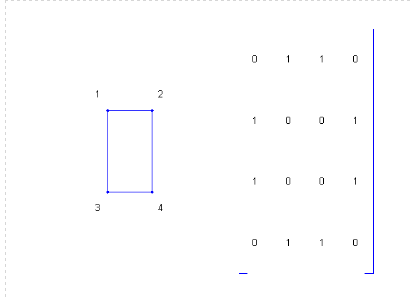这里是巴基球一个半球中的节点，由polygon.subplot（1,1,1）编号的多边形。

子图（1,1,1）;
gplot（B（1:30,1：30），V（1:30，:)，'b-'）;
对于j = 1:30，
文本（V（j，1），V（j，2），int2str（j），'FontSize'，10）;
结束
轴关闭相等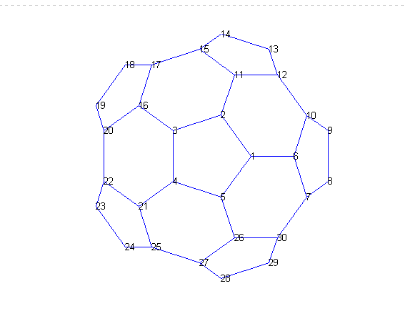为了可视化该半球的邻接矩阵，我们使用SPY函数绘制非零元素的轮廓。注意矩阵是对称的，因为如果节点i连接到节点j，则节点j连接到节点i。
间谍（B（1：30,1：30））
title（'spy（B（1：30,1：30））'）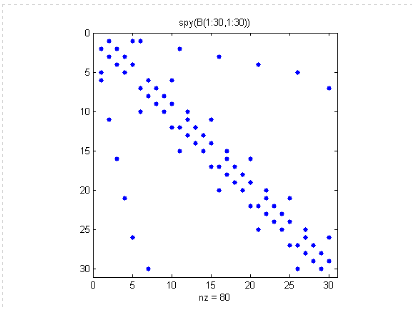现在我们通过将一个半球的编号反映到另一个半球中，将我们的编号方案扩展到整个图形。
[B，V] =巴基;
H =稀疏（60,60）;
k = 31:60;
H（k，k）= B（k，k）;
gplot（B-H，V，'b-'）;
等一下
gplot（H，V，'r-'）;
对于j = 31:60
文本（V（j，1），V（j，2），int2str（j），...
'FontSize'，10，'HorizontalAlignment'，'center'）;
结束
拖延
轴关闭相等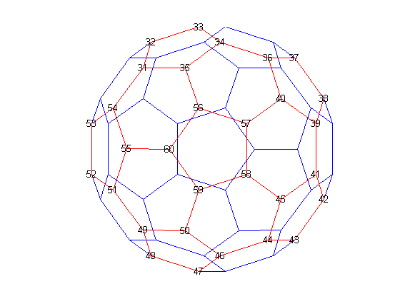最后，这里是一个SPY图的最终稀疏矩阵.
间谍（B）
title（'spy（B）'）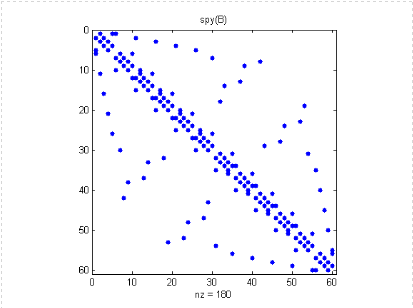在许多有用的图中，每个节点仅连接到几个其他节点。结果，邻接矩阵每行仅包含几个非零条目。本示例显示了SPARSE矩阵在handy.gplot（B-H，V，'b-'）中的一个位置。
gplot（B-H，V，'b-'）;
轴关闭相等
等一下
gplot（H，V，'r-'）;
拖延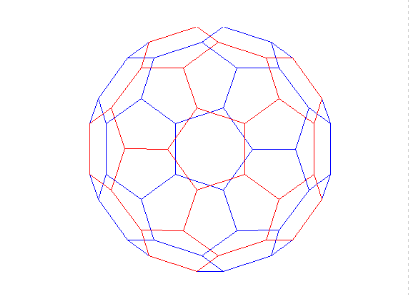demo2 1900年到2000年的美国人口普查数据。
％ 时间间隔
t =（1900：10：2000）';
％人口
p = [75.995 91.972 105.711 123.203 131.669 ...
150.697 179.323 203.212 226.505 249.633 281.422]';
％图
plot（t，p，'bo'）;
轴（[1900 2020 0 400]）;
标题（'美国人口1900-2000'）;
（'Millions'）;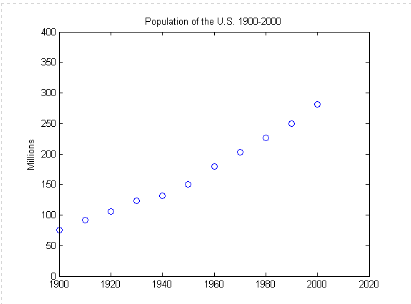你在2010年的人口估计是多少？
p =
75.9950
91.9720
105.7110
123.2030
131.6690
150.6970
179.3230
203.2120
226.5050
249.6330
281.4220
让我们用t中的多项式拟合数据，并使用它外推到t = 2010。多项式中的系数是通过求解涉及11乘11 Vandermonde矩阵的线性方程组获得的，其中元素是缩放时间的幂，n（n），n（j），n（i，j）
s =（t-1950）/ 50;
A = zeros（n）;
A（：，end）= 1;
对于j = n-1：-1：1，A（：，j）= s * A（：，j + 1）结束
通过求解涉及Vandermonde矩阵的最后d + 1列的方程的线性系统，获得适合数据p的度为d的多项式的系数c：A（：nd：n）* c - = pIf d小于10，有比未知数更多的方程，最小二乘解是适当的。如果d等于10，则可以精确求解方程，并且多项式实际内插数据。在任一情况下，系统都用MATLAB的反斜杠运算符求解。这里是立方fit.c = A（：，n-3：n）\ p的系数
c =
1.2629
23.7261
100.3659
155.9043
现在我们从1900年至2010年每年评估多项式，并绘制结果.v =（1900：2020）';
x =（v-1950）/ 50;
w =（2010-1950）/ 50;
y = polyval（c，x）;
z = polyval（c，w）;
等一下
plot（v，y，'k-'）;
plot（2010，z，'ks'）;
text（2010，z + 15，num2str（z））;
拖延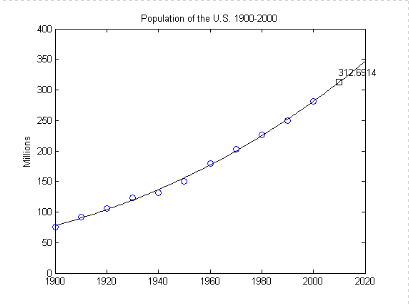将立方拟合与四次方拟合比较。 注意，外推点是非常不同的c = A（：，n-4：n）\ p;
y = polyval（c，x）;
z = polyval（c，w）;
等一下
plot（v，y，'k-'）;
plot（2010，z，'ks'）;
text（2010，z-15，num2str（z））;
拖延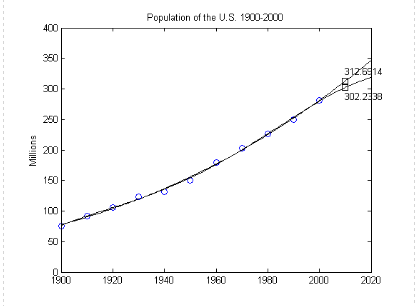随着度的增加，外推变得更加不稳定
plot（t，p，'bo'）; 等一下; 轴（[1900 2020 0 400]）;
colors = hsv（8）; labels = {'data'};
对于d = 1：8
[Q，R] = qr（A（：，n-d：n））;
R = R（1：d + 1，:); Q = Q（：，1：d + 1）;
c = R \（Q'* p）; ％与c = A（：，n-d：n）\ p相同;
y = polyval（c，x）;
z = polyval（c，11）;
plot（v，y，'color'，colors（d，:)）;
标签{end + 1} = ['degree ='int2str（d）];
结束
图例（标签，2）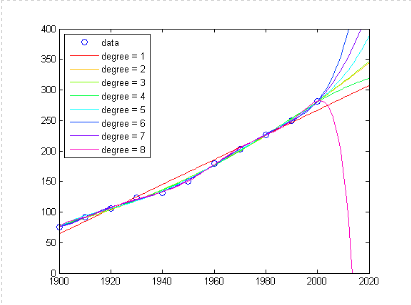展开全文• 一个不错的matlab工具，里面包含了课件以及例程和源程序
• 关于matlab 整数取整问题： <a data-cke-saved-href="https://blog.csdn.net/hjq376247328/article/details/70878110" href="https://blog.csdn.net/hjq376247328/article/details/70878110">...


练习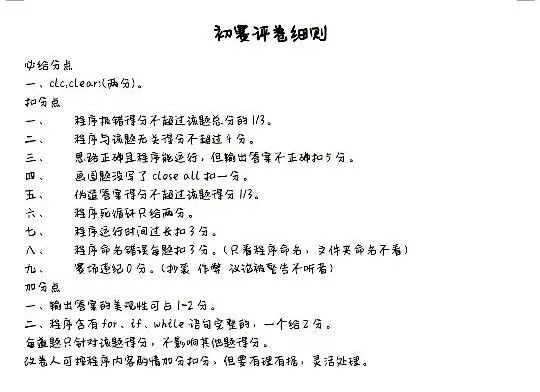%编写程序使任意输入的一个数反转，如输入123456，输出654321
clc,clear;
a = input('输入一个整数\n');
b = 0;
while a ~= 0
b = b * 10 + mod(a,10);
a = fix(a / 10);  %截尾取整数 %% https://blog.csdn.net/hjq376247328/article/details/70878110
end
b


关于matlab 整数取整问题：

<a data-cke-saved-href="https://blog.csdn.net/hjq376247328/article/details/70878110" href="https://blog.csdn.net/hjq376247328/article/details/70878110">https://blog.csdn.net/hjq376247328/article/details/70878110

</a>

%输入一个数，判断是否为质数
clc,clear;
a = input('请输入一个数: '); %输入数字
flag = 0;
% cnt = 0;    %100以内共有25个质数
% for a = 1:100
for i=2:sqrt(a)
if mod(a,i) == 0
flag = 1;
%             cnt = cnt + 1;
break;
end
end
if flag == 1
fprintf('%d不是质数', a);  %输出语句
else
fprintf('%d是质数', a);
end
% end
% cnt

str = input('需要输入的文本','s')  %输入字符串

matlab输入输出：

https://blog.csdn.net/yewuzhitou/article/details/81906379

%三位数的每位数字的立方和为它本身，此数为水仙花数，找到所有的这样的数。
clc,clear;
for i = 100:999
t = i;
a = mod(t,10);
b = mod(fix(t/10),10);
c = mod(fix(t/100),10);
if a^3 + b^3 + c^3 == t
fprintf('%d ', i);
end
endclc,clear;
a = input('请输入一个数：\n');
flag = 0;
aa = a;
while a ~= 0
if a == 1
flag = 1;
break;
end
for i = 2:sqrt(a)
if mod(a,i) == 0
flag = 1;
break;
end
end
a = fix(a/10);
end
if flag == 0
fprintf('%d是特殊质数', aa);
else
fprintf('%d不是特殊质数', aa);
end


%判断是不是回文字符
clc,clear;
str = input('请输入字符串','s');
len = length(str);
flag = 1;
for i=1:fix(len/2)
if str(i) ~= str(len + 1 - i)
flag = 0; %则不是回文
break;
end
end
if flag == 0
fprintf('%s不是回文字符',str);
else
fprintf('%s是回文字符',str);
end



展开全文• ## matlab例题及demo分析

千次阅读 2017-03-11 08:34:42
1.3.4MATLAB提示出错 1.3.5用函数zeros生成全零阵 1.3.6用函数eye生成全零阵 1.3.7矩阵的加减运算 1.3.8两个矩阵的乘法运算 1.3.9矩阵的数乘运算 1.3.10向量的点积 1.3.11向量的...函数 fft
• 1.3-2 输入一个行矩阵   1.3-3 可以分行输入一个行矩阵 ...1.3-4 MATLAB提示出现错误   1.3-5 用函数zeros生成全零阵   1.3-6 用函数eye生成全零阵   1.3-7 矩阵的加减运算
• 1.3.4 Matlab提示出错 1.3.5 用函数zeros生成全零阵 1.3.6 用函数eye生成全零阵 1.3.7 矩阵的加减运算 1.3.8 两个矩阵的乘法运算 1.3.9 矩阵的数乘运算 1.3.10 向量的点积 1.3.11 向量的叉乘 1.3.12 向量...
• 1.3.2输入一个行矩阵 ...1.3.4MATLAB提示出错 1.3.5用函数zeros生成全零阵 1.3.6用函数eye生成全零阵 1.3.7矩阵的加减运算 1.3.8两个矩阵的乘法运算 1.3.9矩阵的数乘运算 1.3.10向
• 2.1-1图像的缩放   2.1-5图像的旋转
• 1.3.2输入一个行矩阵 1.3.3分行输入一个行矩阵 1.3.4MATLAB提示出错 1.3.5用函数zeros生成全零阵 1.3.6用函数eye生成全零阵 1.3.7矩阵的加减运算 1.3.8两个矩阵的乘法运算 1.3.9矩阵的数乘运算 1.3.10向量的点积 ...
• 文章中的例题大多为扎实基础的常规性题目和帮助加深理解的概念辨析题，并适当选取了一些考研数学试题。所选题目难度各异，对于一些难度较大或对理解所学知识有帮助的“经典好题”，我们会详细讲解。阅读更多“高等...
• 则 x k+1 的计算公式为 x k+1= x k -f(x k ) :heavy_division_sign: f’(x k ) （ k=0,1,2…… ） 例题讲解 例： 用牛顿迭代法三次求方程f(x)=x 5 -x 2 +x-30=0, 在区间 [1,3] 中的近似值请详细解答 解： f (1)=-29...
• matlab教学例题代码-例题全部代码.rar 本附件是关于一些matlab基础应用的教学例题代码，还有各种高等数学的内容，希望大家可以借鉴。
• 复制MATLAB例题中提供的engwindemo.c文件，用VS2010打开。本人是用MATLAB打开后，复制到了一个已有的项目及文件中的。 此时就出现一个问题，即VS无法识别engine.h这个头文件。原因是没有在包含目录及库目录里添加...c语言
• 例题：对于a*x^2+b*x+c=0,其中a,b,c需要自己输入。 syms x; a=input("请输入a的值："); b=input("请输入b的值："); c=input("请输入c的值："); % y=a*x.^2+b*x+c; % result=solve(a*x.^2+b*x+c==0,x); %第一种形式...
• ## matlab 画图例题篇

万次阅读 2018-05-17 09:37:44
1、画出[0,2pi] 范围之内的sin曲线syms x&gt;&gt; x=[0:0.01:2*pi];&gt;&gt; plot(sin(x))2、画出间距0.05，[0,2pi] 范围之内的sin曲线的离散图形syms x&... plot(sin(x))3、利用已学知识，画出...
• MATLAB 粒子群算法 本文学习自：Particle Swarm Optimization in MATLAB - Yarpiz Video Tutorial 与《精通MATLAB智能算法》 1. 简介： Particle Swarm Optimization ，粒子群优化算法，常用来找到方程......

# matlab例题matlab 订阅